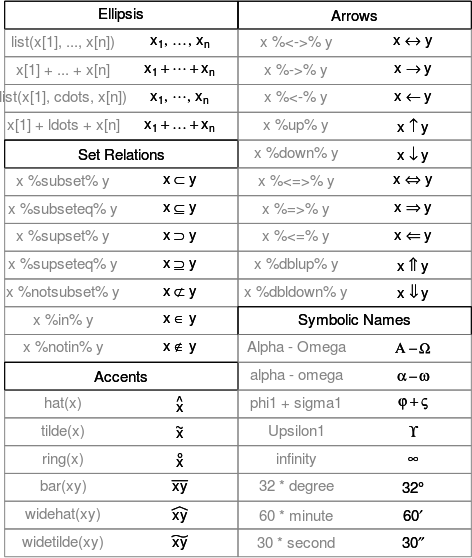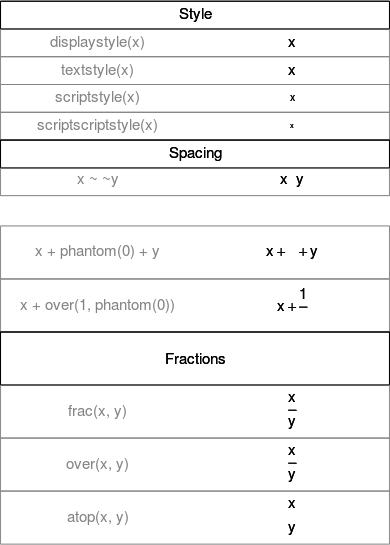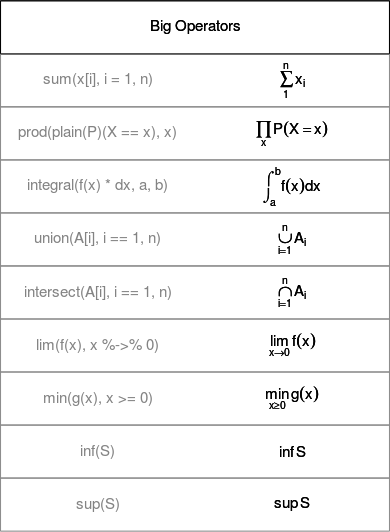# Mathematical Annotation in R

Mathematical Annotation in R | Vistat

Want to write mathematical symbols and expressions in R graphics? You can use an R `expression()` instead of normal text, e.g. `plot(1:10, main = expression(alpha + beta))`. Below is a demo that shows you everything about plotting math in R (it was written by the R Core Team; see `?plotmath` for details):

````demo<span class="p">(</span>plotmath<span class="p">)</span>````

This site uses Akismet to reduce spam. Learn how your comment data is processed.